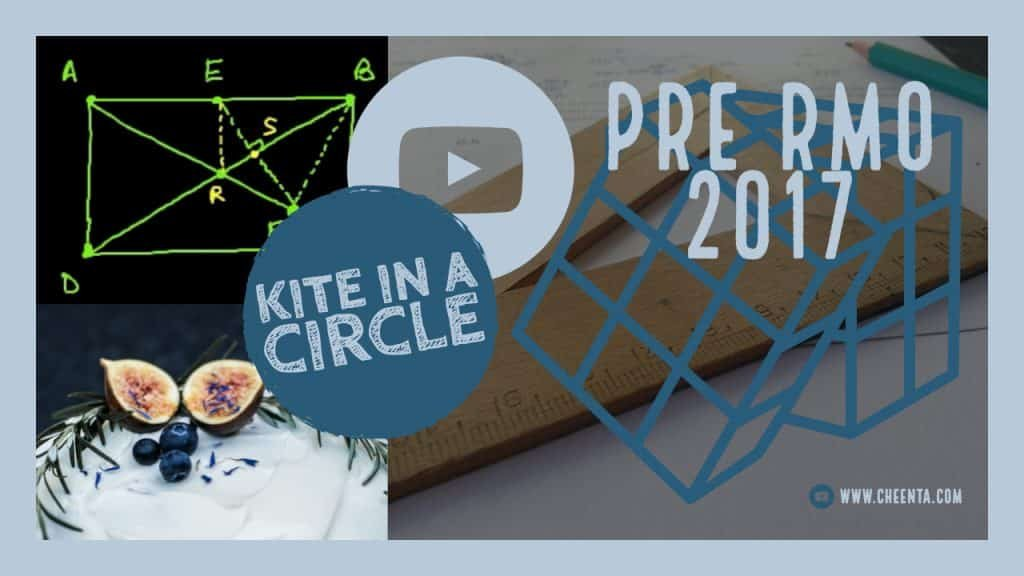How Cheenta works to ensure student success?
Explore the Back-Story

# Pre RMO 2017 Problem 13 Solution - Kite in a CircleCyclic Quadrilaterals are often important objects in a Geometry problem. Recognizing them can lead to a path to the solution. Pre RMO 2017 Problem 13 Solution is a part of our Pre-RMO problem solving series.

Also visit: Math Olympiad Program of Cheenta

## How to recognize cyclic quadrilaterals?

• Opposite angles add up to $\pi$
• Two angles subtended by one segment are equal
• Sum of the product of opposite sides equal product of diagonals

Now look into this problem:

# Part 2

## Part 3

Cyclic Quadrilaterals are often important objects in a Geometry problem. Recognizing them can lead to a path to the solution. Pre RMO 2017 Problem 13 Solution is a part of our Pre-RMO problem solving series.

Also visit: Math Olympiad Program of Cheenta

## How to recognize cyclic quadrilaterals?

• Opposite angles add up to $\pi$
• Two angles subtended by one segment are equal
• Sum of the product of opposite sides equal product of diagonals

Now look into this problem:

# Part 2

## Part 3

This site uses Akismet to reduce spam. Learn how your comment data is processed.

### Knowledge Partner